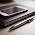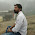Hacker Rank solution for A Very Big Sum in C++

Problem:- Solution of Hackerrank - a very big sum hackerrank solution c++ ora very big sum in java or a very big sum solution or a very big sum solution in C++ or a very big sum hacker rank solution in Java or a very big sum hacker rank solution c++ or a very big sum hacker rank solution c++ or a very big sum program in c or a very big sum solution in c or a very big sum hacker rank solution in python or a very big sum in java or a very big sum hacker rank solution in c or Hacker Rank Solution For a very big sum in C++ or a very big sum in C++ or Hacker Rank solution for a very big sum in C++.

Check This- Hacker rank Solution for C++ Sub Domain Introduction, Classes, STL, Inheritance.

Logic:- a very big sum is similar to an array sum or we can say that both problems are same the only title is different. The same logic applies first take an input from the user and store the input in an array after that take a long long int variable(Cause our array is integer type) and add the array elements and store in long size variable in the end print the sum. See below explanation part.

Explanation:- The size of sum variable should be big for the big size we are taking long long int sum. by using a For Loop add all elements of an array and print the sum. We can do this by using one Loop and By using separate Loops for Both storing elements and Sum of elements of an array.

for(int arr_i = 0;arr_i < n;arr_i++)
{
cin >> arr[arr_i];
sum+=arr[arr_i];
}

cout<<sum;

We got our solution of a problem.

Also check- Geeksforgeeks solution for School, Basic, Easy, Medium, Hard Domain.

Copy the full solution or colored code and paste into the hacker rank editor and click to Run Code if the code runs successfully then click to Submit Code. Before pasting the code into the editor make sure you have chosen c++ editor in the top right drop down option.

Solution:-

#include <cmath>
#include <cstdio>
#include <vector>
#include <iostream>
#include <algorithm>
using namespace std;

int main()
{
int n;
long long int sum=0;

cin >> n;

vector<int> arr(n);

for(int arr_i = 0;arr_i < n;arr_i++)
{
cin >> arr[arr_i];
sum+=arr[arr_i];
}

cout<<sum;
return 0;

}

Output:-

1.What is the function of this line vector arr(n); ?

1.vector arr(n);

2.Hello, Please read vector in C++, Vector is similar to an array in CPP. Here Vector is a Keyword and is the type of vector and arr is the name of array or we can say that vector and in the last (n) is the size of the vector. This is same as an array check both below are same.

int arr[] == vector arr(n);